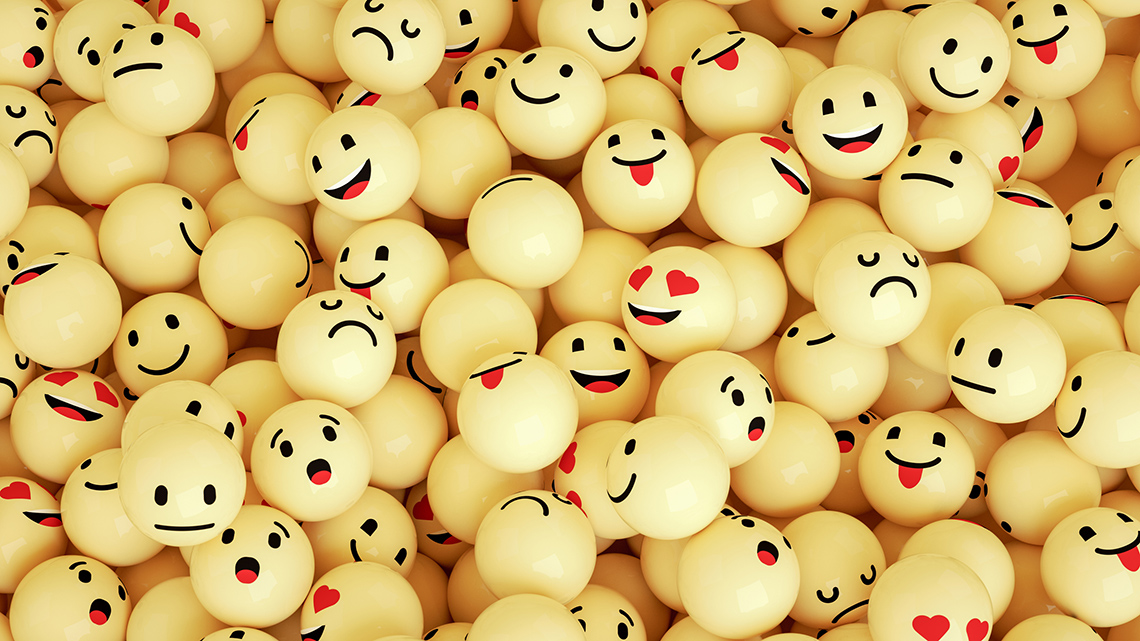# Minds On

## Math equations

Explore the following image of two block towers:

• How are these towers the same?
• How are these towers different?
• Are they equal? How do you know?

Let’s explore another set of towers:

• How are these towers the same?
• How are these towers different?
• Are they equal? How do you know?

### Expressions and equations

For the first two towers, we could record the blocks in the towers like this:

3 red + 2 green for Tower #1, and 4 red + 1 green for Tower #2.

OR

3 + 2 and 4 + 1.

For the second set of towers, we could record the blocks in the towers like this:

3 orange + 4 green + 3 white for Tower #1, and 1 yellow + 7 green + 2 blue for Tower #2.

OR

3 + 4 + 3 and 1 + 7 + 2.

All of the number sentences here are called expressions. What else do we notice about these expressions?

3 + 2 and 4 + 1 are equal.

2 + 4 + 3 and 1 + 7 + 2 are also equal.

They can be written like this:

3 + 2 = 4 + 1

or 2 + 4 + 3 = 1 + 7 + 2

When we see equal expressions on both sides of the equal sign, we call it an equation.

# Action

## Emoji equation

Sometimes equations will have a missing piece. You need to figure out how to make both sides of the equation equal.

### Emoji challenge

Let’s practice! You will now answer the following emoji equation.

Record your ideas in a notebook or a method of your choice. You can use blocks or other manipulatives to help you solve this equation.

# Consolidation

## Patterns in problem-solvingThink about the emoji challenge you just completed and answer these questions:

• How many different ways could the emojis equal nine?
• Have you found all the ways to equal nine?
• How do you know?
• How can you organize your solutions?
• What patterns do you notice?

Record your ideas in a notebook or a method of your choice. Be prepared to share!

## Reflection

How do you feel about what you have learned in this activity? Which of the next four sentences best matches how you are feeling about your learning? Press the button that is beside this sentence.